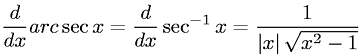Equations > Calculus > Differentiation/Differentals > Derivative of Inverse Secant (Arcsecant)

### Derivative of Inverse Secant (Arcsecant)Latex Code:

MathML Code:

 $\fracd\mathrm{dx}\mathrm{arc}\mathrm{sec}x=\fracd\mathrm{dx}{\mathrm{sec}}^{-1}x=\frac1|x|\sqrt{{x}^{2}-1}$

MathType 5.0: